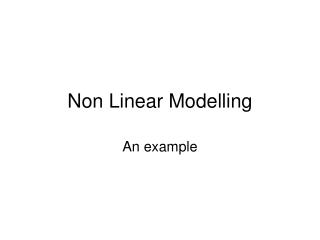DownloadDownload PresentationNon Linear Modelling

# Non Linear Modelling

Download Presentation## Non Linear Modelling

- - - - - - - - - - - - - - - - - - - - - - - - - - - E N D - - - - - - - - - - - - - - - - - - - - - - - - - - -
##### Presentation Transcript

1. Non Linear Modelling An example

2. Background Ace Snackfoods, Inc. has developed a new snack product called Krunchy Bits. Before deciding whether or not to “go national” with the new product, the marketing manager for Krunchy Bits has decided to commission a year-long test market using IRI’s BehaviorScan service, with a view to getting a clearer picture of the product’s potential. The product has now been under test for 24 weeks. On hand is a dataset documenting the number of households that have made a trial purchase by the end of each week. (The total size of the panel is 1499 households.) The marketing manager for Krunchy Bits would like a forecast of the product’s year-end performance in the test market. First, she wants a forecast of the percentage of households that will have made a trial purchase by week 52.

3. Data

4. Approaches to Forecasting Trial • French curve • “Curve fitting”—specify a flexible functional form • fit it to the data, and project into the future. • Inspect the data (see Non Linear Modelling .xls)

5. Proposed Model for this example Y = p0(1 – e–bx) Decreasing returns and saturation. Here: p0 = saturation proportion b = decreasing returns parameter Widely used in marketing.

6. Data

7. Modelled data

8. How well does the model do?

9. How well does the model do – forecasting?

10. Doing the same thing in R NLeg.df=read.csv(file.choose(),header=T) attach(NLeg.df) fit.nls<- nls( propHH ~ p0*(1-exp(-beta*Week )), data = NLeg.df, start = list( p0=.05,beta=.1), trace = TRUE )

11. Doing the same thing in R > fit.nls<- nls( propHH ~ p0*(1-exp(-beta*Week )), + data = NLeg.df, + start = list( p0=.05,beta=.1), + trace = TRUE ) 0.00450657 : 0.05 0.10 0.003392708 : 0.08067993 0.04530598 0.0002417058 : 0.07951665 0.06836061 0.0001274101 : 0.08637640 0.06347303 0.0001261767 : 0.08661768 0.06369747 0.0001261767 : 0.08661641 0.06369891 > > summary(fit.nls) Formula: propHH ~ p0 * (1 - exp(-beta * Week)) Parameters: Estimate Std. Error t value Pr(>|t|) p0 0.086616 0.004462 19.41 2.49e-15 *** beta 0.063699 0.005721 11.13 1.65e-10 *** --- Signif. codes: 0 `***' 0.001 `**' 0.01 `*' 0.05 `.' 0.1 ` ' 1 Residual standard error: 0.002395 on 22 degrees of freedom Correlation of Parameter Estimates: p0 beta -0.9798

12. Different types of Models & Their Interpretations

13. } 1 A Simple Model Y (Sales Level) } b (slope of the salesline) a (sales level when advertising = 0) X (Advertising)

14. Q Phenomena P1: Through Origin P2: Linear Y Y X X P3: Decreasing Returns (concave) P4: Saturation Y Y X X

15. Phenomena P5: Increasing Returns (convex) P6: S-shape Y Y X X P7: Threshold P8: Super-saturation Y Y X X

16. Aggregate Response Models:Linear Model Y = a + bX • Linear/through origin • Saturation and threshold (in ranges)

17. Aggregate Response Models:Fractional Root Model Y = a + bXc c can be interpreted as elasticity when a = 0. Linear, increasing or decreasing returns (depends on c).

18. Aggregate Response Models:Exponential Model Y = aebx; x > 0 Increasing or decreasing returns (depends on b).

19. Xc d + Xc Aggregate Response Models:Adbudg Function Y = b + (a–b) S-shaped and concave; saturation effect. Widely used. Amenable to judgmental calibration.

20. Aggregate Response Models:Multiple Instruments • Additive model for handling multiple marketing instruments Y = af (X1) + bg (X2) Easy to estimate using linear regression.

21. Aggregate Response Models:Multiple Instruments cont’d • Multiplicative model for handling multiple marketing instruments Y = aXb Xc b and c are elasticities. Widely used in marketing. Can be estimated by linear regression. 12